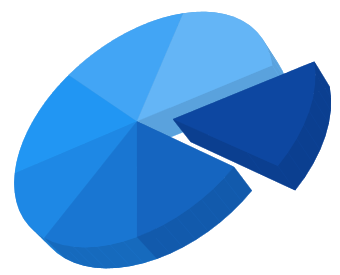# Series in WPF SfChart3D

7 May 202124 minutes to read

ChartSeries is the visual representation of the data. SfChart3D offers eight types of series. Based on your requirements and specifications, any type of Series can be added for data visualization.

• Column
• Bar
• Line
• Scatter
• Area
• Stacking column
• Stacking column 100
• Stacking bar
• Stacking bar
• Pie
• Doughnut

The following APIs are common for the most of the series types:

## Column Charts

`ColumnSeries3D` plots discrete rectangles for the given values.

``````<chart:ColumnSeries3D ItemsSource="{Binding CategoricalData}"
XBindingPath="Year" YBindingPath="Metal"></chart:ColumnSeries3D>``````
``````ColumnSeries3D series = new ColumnSeries3D()
{
ItemsSource = new CategoryDataViewModel().CategoricalData,
XBindingPath = "Year",
YBindingPath = "Metal"
};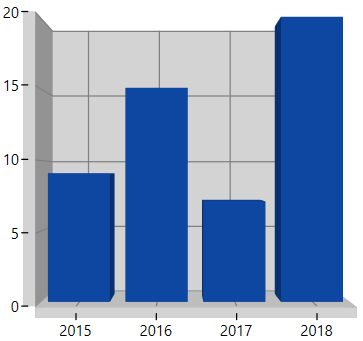## Bar Charts

`BarSeries3D` are similar to column series, excepts its orientation.

``````<chart:BarSeries3D ItemsSource="{Binding CategoricalData}" XBindingPath="Year"
YBindingPath="Plastic"></chart:BarSeries3D>``````
``````BarSeries3D series = new BarSeries3D()
{
ItemsSource = new CategoryDataViewModel().CategoricalData,
XBindingPath = "Year",
YBindingPath = "Plastic"
};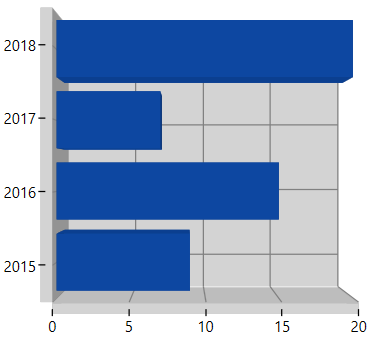### Spacing

`Spacing` property of series is used to decide the width of a segment. `Spacing` value ranges from 0 to 1. The following code illustrates how to set `Spacing` property of the series,

``````<chart:ColumnSeries3D chart:ChartSeriesBase.Spacing="0.6" ItemsSource="{Binding CategoricalData}"
XBindingPath="Year" YBindingPath="Plastic"></chart:ColumnSeries3D>``````
``````ColumnSeries3D series = new ColumnSeries3D()
{
ItemsSource = new CategoryDataViewModel().CategoricalData,
XBindingPath = "Year",
YBindingPath = "Plastic"
};
ChartSeriesBase.SetSpacing(series, 0.6);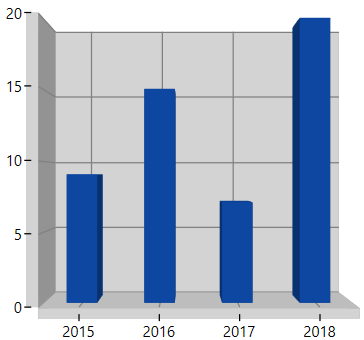Segment Spacing

`SegmentSpacing` property is used to set the spacing among the segments, when multiple series are added in chart. Its value ranges from 0 to 1. The following code illustrates how to use the `SegmentSpacing` property in series,

``````<chart:ColumnSeries3D SegmentSpacing="0.6" ItemsSource="{Binding CategoricalData}"
XBindingPath="Year" YBindingPath="Plastic"></chart:ColumnSeries3D>

<chart:ColumnSeries3D SegmentSpacing="0.6" ItemsSource="{Binding CategoricalData}"
XBindingPath="Year" YBindingPath="Iron"></chart:ColumnSeries3D>``````
``````ColumnSeries3D series = new ColumnSeries3D()
{
ItemsSource = new CategoryDataViewModel().CategoricalData,
XBindingPath = "Year",
YBindingPath = "Plastic"
SegmentSpacing = 0.6,
};

ColumnSeries3D series1 = new ColumnSeries3D()
{
ItemsSource = new CategoryDataViewModel().CategoricalData,
XBindingPath = "Year",
YBindingPath = "Iron"
SegmentSpacing = 0.6,
};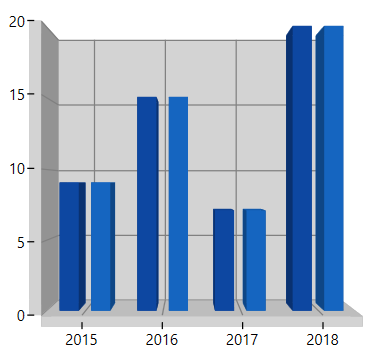## Line Charts

`LineSeries3D` join points on a plot by straight lines, showing data trends at equal intervals.

``````<chart:LineSeries3D ItemsSource="{Binding CategoricalData}" XBindingPath="Year"
YBindingPath="Metal"></chart:LineSeries3D>``````
``````LineSeries3D line = new LineSeries3D()
{
ItemsSource = new CategoryDataViewModel().CategoricalData,
XBindingPath = "Year",
YBindingPath = "Metal"
};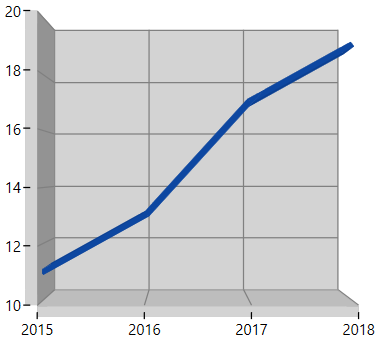## Scatter Chart

`ScatterSeries3D` will represent each point by a Rectangle with equal size.

This size can be defined by using below properties.

``````<chart:ScatterSeries3D  ItemsSource="{Binding DataPoints}"
XBindingPath="Year" YBindingPath="Germany"></chart:ScatterSeries3D>``````
``````ScatterSeries3D series = new ScatterSeries3D()
{
ItemsSource = new ViewModel().DataPoints,
XBindingPath = "Year",
YBindingPath = "Germany"
};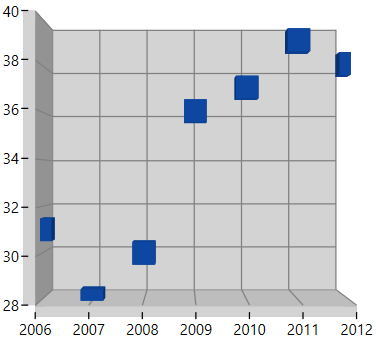## Area Chart

`AreaSeries3D` is rendered using a collection of line segments connected to form a closed loop area, filled with the specified color.

``````<chart:AreaSeries3D ItemsSource="{Binding CategoricalData}"  XBindingPath="Year"
YBindingPath="Plastic"></chart:AreaSeries3D>``````
``````AreaSeries3D series = new AreaSeries3D()
{
ItemsSource = new CategoryDataViewModel().CategoricalData,
XBindingPath = "Year",
YBindingPath = "Plastic"
};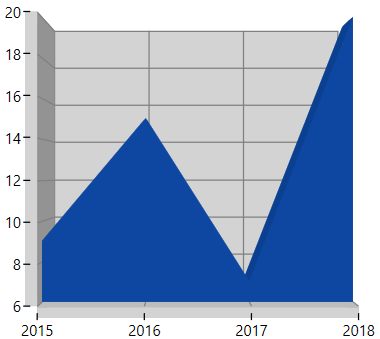## Stacking Charts

### Stacking Column

`StackingColumnSeries3D` resembles multiple types of ColumnSeries3D. Each series is vertically stacked one above the other. When there is only one series, then it is ColumnSeries.

The following code example illustrates how to use StackingColumnSeries3D:

``````<chart:StackingColumnSeries3D ItemsSource="{Binding CategoricalData}"  XBindingPath="Year"
YBindingPath="Plastic"></chart:StackingColumnSeries3D>

<chart:StackingColumnSeries3D ItemsSource="{Binding CategoricalData}"  XBindingPath="Year"
YBindingPath="Iron"></chart:StackingColumnSeries3D>

<chart:StackingColumnSeries3D ItemsSource="{Binding CategoricalData}"  XBindingPath="Year"
YBindingPath="Metal"></chart:StackingColumnSeries3D>``````
``````StackingColumnSeries3D stack1 = new StackingColumnSeries3D()
{
ItemsSource = new CategoryDataViewModel().CategoricalData,
XBindingPath = "Year",
YBindingPath = "Plastic"
};

StackingColumnSeries3D stack2 = new StackingColumnSeries3D()
{
ItemsSource = new CategoryDataViewModel().CategoricalData,
XBindingPath = "Year",
YBindingPath = "Iron"
};

StackingColumnSeries3D stack3 = new StackingColumnSeries3D()
{
ItemsSource = new CategoryDataViewModel().CategoricalData,
XBindingPath = "Year",
YBindingPath = "Metal"
};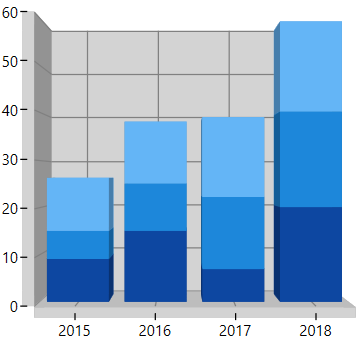### Stacking Column 100

`StackingColumn100Series3D` resembles StackingColumnSeries3D but the cumulative portion of each stacked element always comes to a total of 100%.

The following code example illustrates how to use StackingColumn100Series3D:

``````<chart:StackingColumn100Series3D ItemsSource="{Binding CategoricalData}"  XBindingPath="Year"
YBindingPath="Plastic"></chart:StackingColumn100Series3D>

<chart:StackingColumn100Series3D Interior="Brown" ItemsSource="{Binding CategoricalData}"  XBindingPath="Year" YBindingPath="Iron"></chart:StackingColumn100Series3D>``````
``````StackingColumn100Series3D stack1 = new StackingColumn100Series3D()
{
ItemsSource = new CategoryDataViewModel().CategoricalData,
XBindingPath = "Year",
YBindingPath = "Plastic"
};

StackingColumn100Series3D stack2 = new StackingColumn100Series3D()
{
ItemsSource = new CategoryDataViewModel().CategoricalData,
XBindingPath = "Year",
YBindingPath = "Iron",
Interior=new SolidColorBrush(Colors.Brown)
};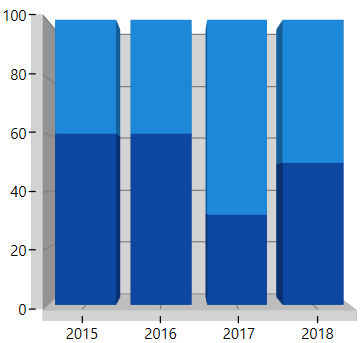### Stacking Bar

`StackingBarSeries3D` is a multiple series type of BarSeries3D. Each BarSeries3D is then stacked horizontally, side by side to each other. When there exists only one series, it resembles a simple BarSeries3D.

The following code example illustrates how to use StackingBarSeries3D:

``````<chart:StackingBarSeries3D ItemsSource="{Binding CategoricalData}"  XBindingPath="Year"
YBindingPath="Plastic"></chart:StackingBarSeries3D>

<chart:StackingBarSeries3D ItemsSource="{Binding CategoricalData}"  XBindingPath="Year"
YBindingPath="Iron"></chart:StackingBarSeries3D>

<chart:StackingBarSeries3D ItemsSource="{Binding CategoricalData}"  XBindingPath="Year"
YBindingPath="Metal"></chart:StackingBarSeries3D>``````
``````StackingBarSeries3D stack1 = new StackingBarSeries3D()
{
ItemsSource = new CategoryDataViewModel().CategoricalData,
XBindingPath = "Year",
YBindingPath = "Plastic"
};

StackingBarSeries3D stack2 = new StackingBarSeries3D()
{
ItemsSource = new CategoryDataViewModel().CategoricalData,
XBindingPath = "Year",
YBindingPath = "Iron"
};

StackingBarSeries3D stack3 = new StackingBarSeries3D()
{
ItemsSource = new CategoryDataViewModel().CategoricalData,
XBindingPath = "Year",
YBindingPath = "Metal"
};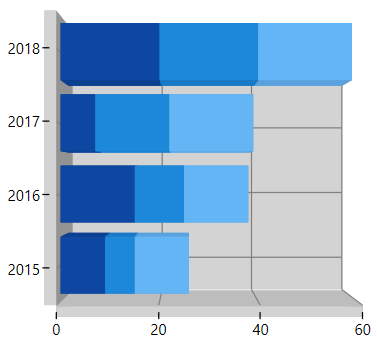### Stacking Bar 100

`StackingBar100Series3D` resembles a StackingBarSeries3D. StackingBar100Series3D displays multiple series as stacked bars and the cumulative portion of each stacked element is always 100%.

The following code example illustrates how to use StackingBar100Series3D:

``````<chart:StackingBar100Series3D ItemsSource="{Binding CategoricalData}"  XBindingPath="Year"
YBindingPath="Plastic"></chart:StackingBar100Series3D>

<chart:StackingBar100Series3D ItemsSource="{Binding CategoricalData}"  XBindingPath="Year"
YBindingPath="Iron"></chart:StackingBar100Series3D>``````
``````StackingBar100Series3D stack1 = new StackingBar100Series3D()
{
ItemsSource = new CategoryDataViewModel().CategoricalData,
XBindingPath = "Year",
YBindingPath = "Plastic"
};

StackingBar100Series3D stack2 = new StackingBar100Series3D()
{
ItemsSource = new CategoryDataViewModel().CategoricalData,
XBindingPath = "Year",
YBindingPath = "Iron"
};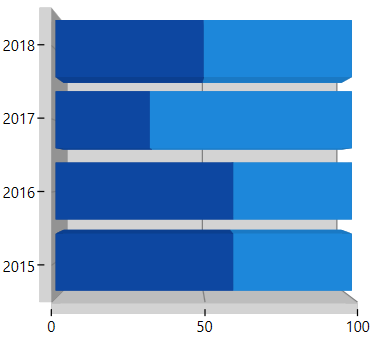## Pie Chart

`PieSeries3D` is divided into sectors, illustrating numerical proportion.

The following code example illustrates the PieSeries3D.

``````<chart:PieSeries3D ItemsSource="{Binding CategoricalData}"  XBindingPath="Year"
YBindingPath="Iron"></chart:PieSeries3D>``````
``````PieSeries3D series = new PieSeries3D()
{
ItemsSource = new CategoryDataViewModel().CategoricalData,
XBindingPath = "Year",
YBindingPath = "Iron"
};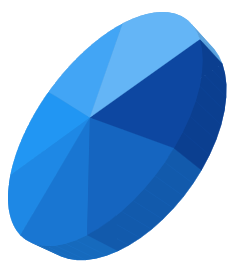## Doughnut Chart

`DoughnutSeries3D` is similar to PieSeries. It is used to show the relationship between parts of data and whole data.

The DoughnutSeries3D can be added to chart as in below code example:

``<chart:DoughnutSeries3D ItemsSource="{Binding CategoricalData}"  XBindingPath="Year"                        YBindingPath="Iron"> </chart:DoughnutSeries3D>``
``````DoughnutSeries3D series = new DoughnutSeries3D()
{
ItemsSource = new CategoryDataViewModel().CategoricalData,
XBindingPath = "Year",
YBindingPath = "Iron"
};### Coefficient

The `DoughnutCoefficient` and `CircleCoefficient` property to define the inner circle of Doughnut and Pie Charts.

``````<chart:DoughnutSeries3D ItemsSource="{Binding CategoricalData}" DoughnutCoefficient="0.5"
XBindingPath="Year" YBindingPath="Iron"> </chart:DoughnutSeries3D>``````
``````DoughnutSeries3D series = new DoughnutSeries3D()
{
ItemsSource = new CategoryDataViewModel().CategoricalData,
XBindingPath = "Year",
YBindingPath = "Iron",
DoughnutCoefficient=0.5
};### Semi Pie and Doughnut

By using custom `StartAngle` and `EndAngle` properties, you can draw pie series in different shapes such as semi or quarter circular series.

``````<chart:DoughnutSeries3D StartAngle="180" EndAngle="360" ItemsSource="{Binding CategoricalData}"
DoughnutCoefficient="0.7" XBindingPath="Year" YBindingPath="Iron"> </chart:DoughnutSeries3D>``````
``````DoughnutSeries3D series = new DoughnutSeries3D()
{
ItemsSource = new CategoryDataViewModel().CategoricalData,
XBindingPath = "Year",
YBindingPath = "Iron",
DoughnutCoefficient=0.7,
StartAngle=180,
EndAngle=360
};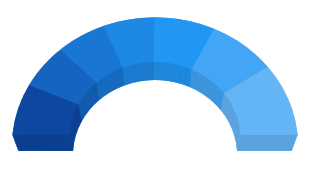Pie

``````<chart:PieSeries3D StartAngle="180" EndAngle="360" ItemsSource="{Binding CategoricalData}"
CircleCoefficient="0.7" XBindingPath="Year" YBindingPath="Iron"> </chart:DoughnutSeries3D>``````
``````PieSeries3D series = new PieSeries3D()
{
ItemsSource = new CategoryDataViewModel().CategoricalData,
XBindingPath = "Year",
YBindingPath = "Iron",
CircleCoefficient=0.7,
StartAngle=180,
EndAngle=360
};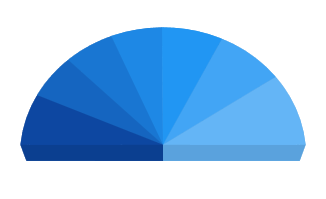## Dynamic explode

This feature allows users to explode a particular segment in a circular series using `ExplodeOnMouseClick`. This can also be achieved by setting the `ExplodeIndex` or `ExplodeAll` property.

The following code example illustrates how to enable dynamic explode for circular series, for data please refer series category in 3D charts.

``````<chart:SfChart3D EnableRotation="True"  Tilt="-30" Rotation="45"
Depth="30" PerspectiveAngle="90" Width="500" Height="500">

<!--PrimaryAxis-->
<chart:SfChart3D.PrimaryAxis>